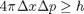Constants

Users have occasionally asked how to create and use constants in MATLAB. Here's one such example. In some cases, they would like to create a constant that other users or programs can't change. Unlike programming languages such as C, MATLAB doesn't have a declaration that enables this. Instead, we have constants, such as pi, defined as functions. When they get used once in a function, their value can't then be overwritten elsewhere in that function. You can use this same technique to make your own constant values.

Motivation

Suppose you're doing some calculations in quantum physics and you need to use Planck's constant h:Solutions

There is no syntax in MATLAB for constant definition. You can place the value for h in each M-file that needs to use it. This becomes both tedious and potentially very error-prone, especially if you type as poorly as I sometimes do.

Alternatively, you can create a function M-file, such as this one

dbtype planck
1     function h = planck
2     %Planck Planck's constant.
3     h = 6.626068e-34; % Units are m^2 kg / s

and use it when you need Planck's constant. If instead you have a constant value that needs to be computed, you can store it in the M-file in a persistent variable and only calculate it the first time the function gets called. This is essentially the way pi works in MATLAB except its value is computed once at startup time and then is available throughout your MATLAB session.

Starting in R14 (MATLAB 7), you can't use a name in a function as both a variable and function so people can't overwrite the M-file version, provided they call your function first. E.g., this code generates a runtime error because of planck being an M-file and then trying to be a variable:

dbtype quantum
% and here's the error you would see
try
quantum
catch
err = lasterror;
disp(err.message)
end
1     function y = quantum(x)
2     %Quantum  Very fake quantum calculation.
3     % Run the function.
4     h = planck;
5     % Now try to place the result in a variable and update it.
6     planck = planck+1;
7     % Do some calculation.
8     y = x*h + planck;
9

Error: File: H:\Documents\LOREN\MyJob\Art of MATLAB\quantum.m Line: 6 Column: 1
"planck" previously appeared to be used as a function or command,
conflicting with its use here as the name of a variable.
A possible cause of this error is that you forgot to initialize the
variable, or you have initialized it implicitly using load or eval.

You also get an M-Lint message in the editor in quantum. Running mlint on quantum, we see a message telling us that we are using the name planck apparently before it is defined.

mlintMsg = mlint('quantum')
mlintMsg =
message: 'The variable 'planck' can apparently be used before it is defined.'
line: 4
column: [5 10]

Since mlint currently performs a static analysis of M-file code, without using information about the rest of the MATLAB environment, including the path, the analysis thinks that a variable is being used here since it's set on the left-hand side, but then it sees that we are using that variable on line 4, before we have a value for it.

What's not solved by placing constants in functions?

Some users would like to ensure that certain inputs to functions not be overwritten during the function execution. Currently there is not a bullet-proof way to guarantee this in MATLAB. Is that something you miss from other languages? Have you had uses for constants in your code? And have you been able to protect them adequately using the technique I outlined here, or perhaps some other one? I'd love to hear more about what needs you have regarding dealing with constants in MATLAB.

Published with MATLAB® 7.3

|